# 【Python数据科学快速入门系列 | 06】Matplotlib数据可视化基础入门(一)# 《Python数据科学快速入门系列》快速导航：

• 【Python数据科学快速入门系列 | 01】Numpy初窥——基础概念
• 【Python数据科学快速入门系列 | 02】创建ndarray对象的十多种方法
• 【Python数据科学快速入门系列 | 03】玩转数据摘取：Numpy的索引与切片
• 【Python数据科学快速入门系列 | 04】Numpy四则运算、矩阵运算和广播机制的爱恨情仇
• 【Python数据科学快速入门系列 | 05】常用科学计算函数
• 【Python数据科学快速入门系列 | 06】Matplotlib数据可视化基础入门

• ### 文章目录

• 《Python数据科学快速入门系列》快速导航：
• 前言
• 1. Matplotlib简介
• 2. Matplotlib的安装
• 3. Matplotlib的基础使用
• 3.1 第一个Matplot例子：绘制折线图
• 3.2 编码风格
• 3.2 绘图参数详解
• 3.2.1 Figure画布
• 3.2.2 Axes绘图区域与Axis坐标轴
• 3.2.3 输入数据的类型
• 3.2.4 绘图样式
• 3.2.4.1 标准表示
• 3.2.4.2 简写表示

• 写在开始：

• 博客简介：专注AIoT领域，追逐未来时代的脉搏，记录路途中的技术成长！
• 博主社区：AIoT机器智能, 欢迎加入！
• 专栏简介：从0到1掌握数据科学常用库Numpy、Matploblib、Pandas。
• 面向人群：AI初级学习者
• # 前言

本文概述了matplotlib是什么，能做什么，怎么做的问题，是一篇matplotlib数据可视化入门文章，对于matplotlib的基础功能做了一个整体的使用说明。包含绘制第一个图表、绘图编程风格、Figure画布、axes绘图区，绘图样式等内容。

# 1. Matplotlib简介

Matplotlib是一个数据可视化综合绘图库，python三剑客(Numpy、Matplotlib、Pandas)之一，用于创建静态图、动态图和Python中的交互式可视化图像。

只需几行代码就可以生成图表，直方图，功率谱，条形图，误差图，散点图等

说到数据可视化，我们为什么需要数据可视化？

如果将文本数据与图表数据相比较，人类的思维模式更适合于理解后者，原因在于图表数据更加直观且形象化，它对于人类视觉的冲击更强，这种使用图表来表示数据的方法被叫做数据可视化。

举个简单的例子：给你一只股票的分时数据，你认为一行行的数据直观呢，还是K线图直观呢？

# 2. Matplotlib的安装

有2种主流的安装方式：

• 第一种直接安装Anaconda就可以自动安装matplotlib库，可以参考博主之前的文章:Python零基础快速入门系列|01】人工智能序章：开发环境搭建Anaconda+VsCode+JupyterNotebook(零基础启动)

• 第二种直接使用命令安装

• ``````pip install matplotlib
``````

``````conda install matplotlib
``````

# 3. Matplotlib的基础使用

## 3.1 第一个Matplot例子：绘制折线图

``````#引入matplotlib.pyplot绘图库
from matplotlib import pyplot as plt

#创建一个只有单个子图的画布
fig,ax=plt.subplots()#Create a figure containing a single axes.

#4个坐标点的坐标分别是(1,1),(2,4),(3,2),(4,3)
ax.plot([1,2,3,4],[1,4,2,3]);#Plot some data on the axes.

plt.show()
``````可以看到仅仅4行代码就生成了非常好看的折线图，C语言开发工程师已经哭晕…

那么它到底怎么做的呢？

• 首先创建了一个画布fig
• 然后创建了一个绘图区域ax(axes)，这个绘图区域有2个坐标轴axis，分别在横轴和纵轴。
• 然后绘图区域对象ax调用了方法plot绘制了4个坐标点，形成折线图。
• ## 3.2 编码风格

有人可能注意到我们在网上看到的绘图代码好像不是这样的，直接使用plt就可以绘制了，就像这样：

``````#引入matplotlib.pyplot绘图库
from matplotlib import pyplot as plt

#4个坐标点的坐标分别是(1,1),(2,4),(3,2),(4,3)
plt.plot([1,2,3,4],[1,4,2,3]);#Plot some data on the axes. b

plt.show()
``````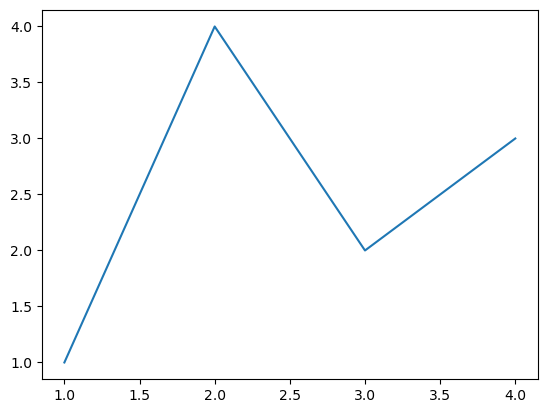从结果来看，是一模一样的，好像更方便，仅仅3行代码就可以了。

第二种绘制方法绘制过程如下：

• 隐式创建一个画布，并创建一个绘图区域
• 然后绘制4个坐标点，绘制折线图
• 其实从编码风格来说，第一种是面向对象的编码风格，第二种是pyplot风格：依靠pyplot自动创建和管理图形和轴，并使用pyplot函数进行绘图。

一般来说，我们建议使用OO风格，特别是对于复杂的绘图，以及旨在作为更大项目的一部分重用的函数和脚本。但是，pyplot样式可以非常方便地进行快速交互工作。

## 3.2 绘图参数详解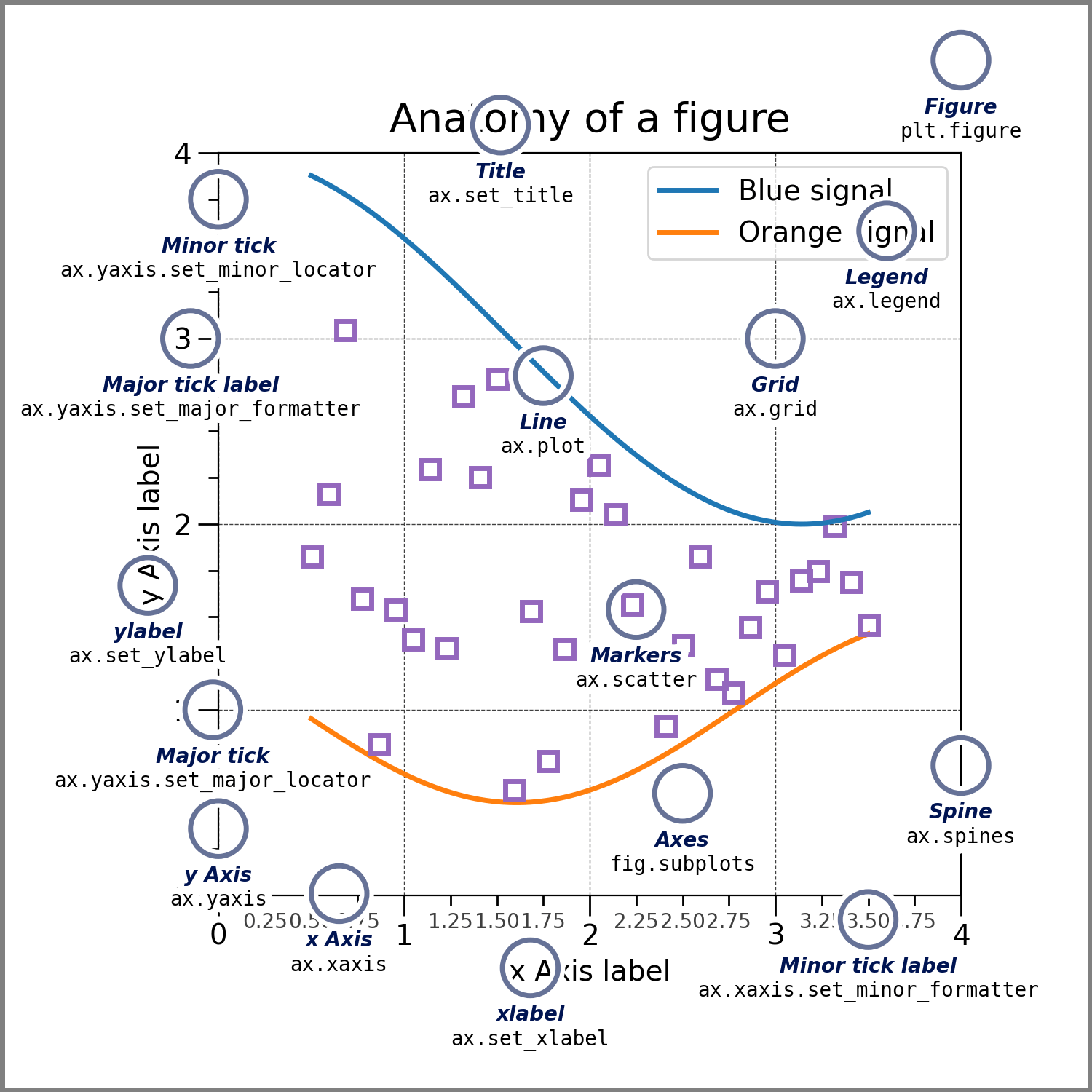### 3.2.1 Figure画布

首先创建的就是画布，创建画布的方式有多种

``````from matplotlib import pyplot as plt

#创建空白画布
fig = plt.figure()#an empty figure with no Axes
plt.show()
``````
``````<Figure size 640x480 with 0 Axes>
``````
``````from matplotlib import pyplot as plt

#创建单个绘图区域的画布
fig,ax=plt.subplots()#a figure with a single Axes
plt.show()
````````````from matplotlib import pyplot as plt

#创建2x2四个绘图区域的画布
fig,axs=plt.subplots(2,2)#a figure with a 2x2 grid of Axes
plt.show()
``````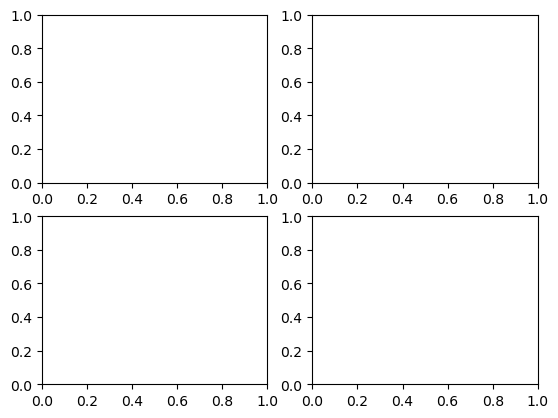### 3.2.2 Axes绘图区域与Axis坐标轴

一个画布可以包含多个绘图区域，如上面的例子，一个画布包含4个绘图区域，每个绘图区域由2个(2D)或3个(3D)坐标轴组成。看下面的结构图的直观展示：### 3.2.3 输入数据的类型

绘图函数需要 numpy.array 或 numpy.ma.masked_array 作为输入，或者可以传递给 numpy.asarray 的对象转换。

x, y array-like or scalar

### 3.2.4 绘图样式

#### 3.2.4.1 标准表示

• color

支持颜色英文名称和十六进制颜色代码，例如black和#000000

• linewidth

浮点类型

• linestyle

• linestyle description
`'-'` or `'solid'` 实线
`'--'` or `'dashed'` 杠虚线
`'-.'` or `'dashdot'` 点杠虚线
`':'` or `'dotted'` 点虚线
`'none'`, `'None'`, `' '`, or `''`
• marker
• marker样式非常多，更多样式参考官方文档:https://matplotlib.org/stable/api/markers_api.html#module-matplotlib.markers

marker symbol description
`"."`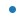point
`","`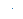pixel
`"o"`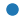circle
`"v"`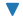triangle_down
`"^"`triangle_up
`"<"`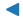triangle_left
`">"`triangle_right
`"1"`tri_down
`"2"`tri_up
`"3"`tri_left
`"4"`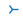tri_right
`"8"`octagon
`"s"`square
`"p"`pentagon
`"P"`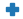plus (filled)
`"*"`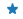star
`"h"`hexagon1
`"H"`hexagon2
`"+"`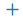plus
`"x"`x
`"X"`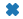x (filled)
`"D"`diamond
`"d"`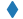thin_diamond
`"|"`vline
`"_"`hline
``````"""
曲线样式例子1
"""

import matplotlib as mpl
import matplotlib.pyplot as plt
import numpy as np

fig, ax = plt.subplots(figsize=(8, 5))
# 生成0~2π之间的等差数列，数据元素为30个
x = np.linspace(0, 2*np.pi, 30)
y1 = np.cos(x)
y2 = np.sin(x)
y3 = np.cos(2*x)
y4 = np.sin(2*x)
# 绘制余弦曲线，颜色为黑色，线宽3，线样式为杠虚线，点标记为小三角
ax.plot(x, y1, color='#000000', linewidth=3, linestyle='--', marker='^')
# 绘制正弦函数曲线，颜色为橙色，线宽2，点标记为星号
l, = ax.plot(x, y2, color='orange', linewidth=2, marker='*')
# 单独设置线样式为点虚线
l.set_linestyle(':')
# 绘制2x余弦函数曲线，线宽为8，线样式为杠点虚线
ax.plot(x, y3, color='red', linewidth=6, linestyle='-.')
# 绘制2x正弦函数曲线，颜色为绿色，线宽为1，线样式为实线
ax.plot(x, y4, color='green', linewidth=2, linestyle='-')
plt.show()

``````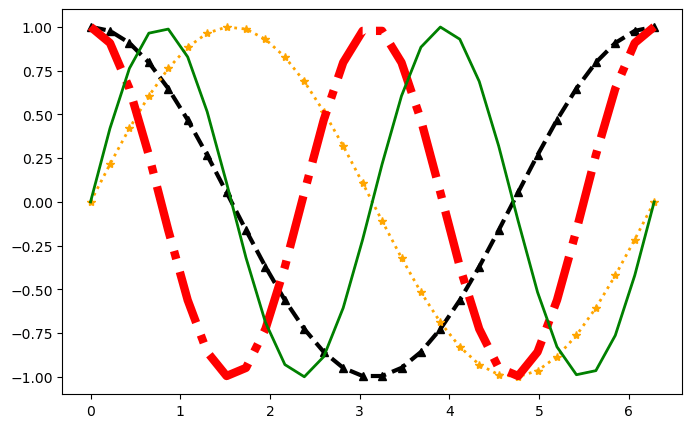#### 3.2.4.2 简写表示

除了上面的设置方式之外，还有一种简写设置方式。

``````plot([x], y, [fmt], *, data=None, **kwargs)
``````
``````fmt = '[marker][line][color]'
或
fmt = '[color][marker][line]'
``````

fmt的内容本身没有限定顺序，可以自由组合。

line指的是line_style，marker和line_style的取值和上面的表描述是一样的，颜色代码简写表示如下：

颜色

支持的颜色缩写是单字母代码

特点 颜色
`'b'` 蓝色的
`'g'` 绿色
`'r'` 红色的
`'c'` 青色
`'m'` 品红
`'y'` 黄色
`'k'` 黑色的
`'w'` 白色的
``````import matplotlib as mpl
import matplotlib.pyplot as plt
import numpy as np

# 生成0~2π之间的等差数列，数据元素为30个
x = np.linspace(0, 2*np.pi, 30)
y1 = np.cos(x)
y2 = np.sin(x)
y3 = np.cos(2*x)

# g-green,o-cycle圆点标记，--为杠杠虚线，其它样式需要单独指定
plt.plot(x, y1, 'go--', linewidth=2, markersize=6)
# c-青色，^-三角标志，:为点虚线
plt.plot(x, y2, 'c^:', linewidth=2, markersize=3)
# b-blue，*-star标志，-.为杠点虚线
plt.plot(x, y3, 'b*-.', linewidth=2, markersize=3)
``````
``````[<matplotlib.lines.Line2D at 0x7fd1e088b6a0>]
``````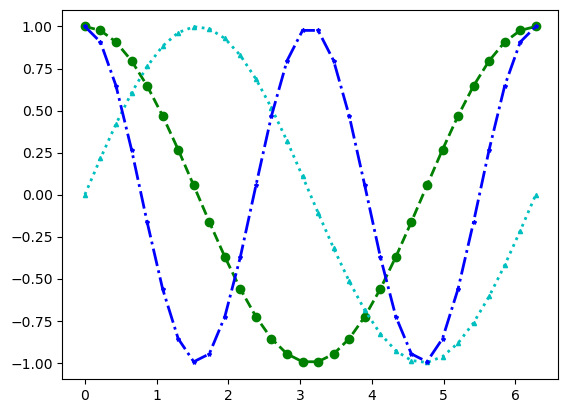未完待续，后续详见下一篇文章：
【Python数据科学快速入门系列 | 06】Matplotlib数据可视化基础入门(二)

— 博主热门专栏推荐 —

• Python零基础快速入门系列
• 深入浅出i.MX8企业级开发实战系列
• MQTT从入门到提高系列
• 物体检测快速入门系列
• 自动驾驶模拟器AirSim快速入门
• 安全利器SELinux入门系列
• Python数据科学快速入门系列
•